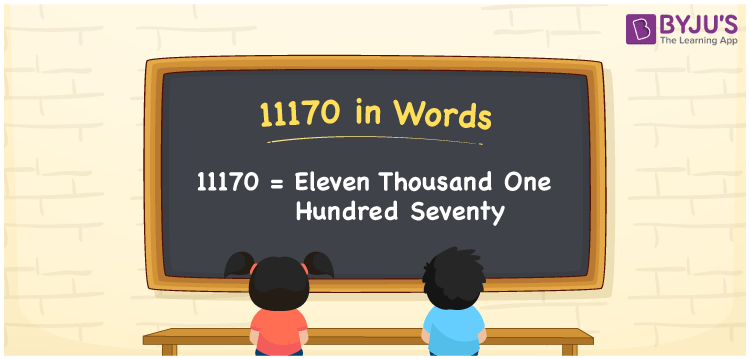# 11170 in words

11170 in words is written as Eleven Thousand One Hundred and Seventy. In 11170, first 1 has a place value of ten thousand, second 1 has a place value of thousand, third 1 has the place value of hundred and 7 is in the place value of ten. The article on Place Value gives more information. The number 11170 is used in expressions that relate to money, distance, length, social media views, and many more. For example, “The pdf format of that story book has exactly Eleven Thousand One Hundred and Seventy words.”

 11170 in words Eleven Thousand One Hundred and Seventy Eleven Thousand One Hundred and Seventy in Numbers 11170

## 11170 in English Words## How to Write 11170 in Words?

We can convert 11170 to words using a place value chart. This can be done as follows. The number 11170 has 5 digits, so let’s make a chart that shows the place value up to 5 digits.

 Ten thousand Thousands Hundreds Tens Ones 1 1 1 7 0

Thus, we can write the expanded form as:

1 × Ten thousand + 1 × Thousand + 1 × Hundred + 7 × Ten + 0 × One

= 1 × 10000 + 1 × 1000 + 1 × 100 + 7 × 10 + 0 × 1

= 11170.

= Eleven Thousand One Hundred and Seventy.

11170 is the natural number that is succeeded by 11169 and preceded by 11171.

11170 in words – Eleven Thousand One Hundred and Seventy.

Is 11170 an odd number? – No.

Is 11170 an even number? – Yes.

Is 11170 a perfect square number? – No.

Is 11170 a perfect cube number? – No.

Is 11170 a prime number? – No.

Is 11170 a composite number? – Yes.

## Solved Example

1. Write the number 11170 in expanded form

Solution: 1 x 10000 + 1 x 1000 + 1 x 100 + 7 x 10 + 0 x 1

Or Just 1 x 10000 + 1 x 1000 + 1 x 100 + 7 x 10

We can write 11170 = 10000 + 1000 + 100 + 70 + 0

= 1 x 10000 + 1 x 1000 + 1 x 100 + 7 x 10 + 0 x 1.

## Frequently Asked Questions on 11170 in words

Q1

### How to write the number 11170 in words?

11170 in words is written as Eleven Thousand One Hundred and Seventy.
Q2

### Is 11170 divisible by 3?

No. 11170 is not divisible by 3.
Q3

### Is 11170 a prime number?

No. 11170 is not a prime number.